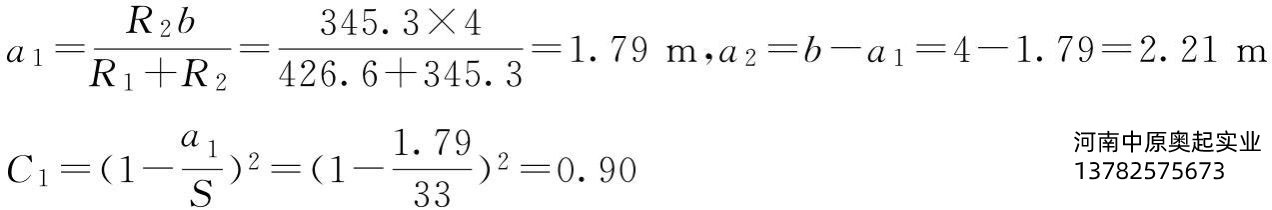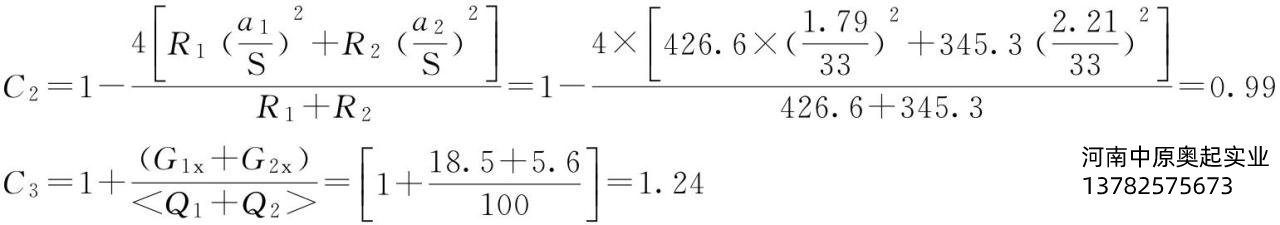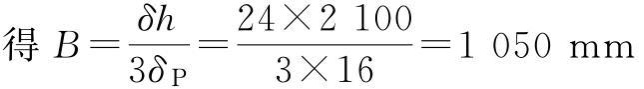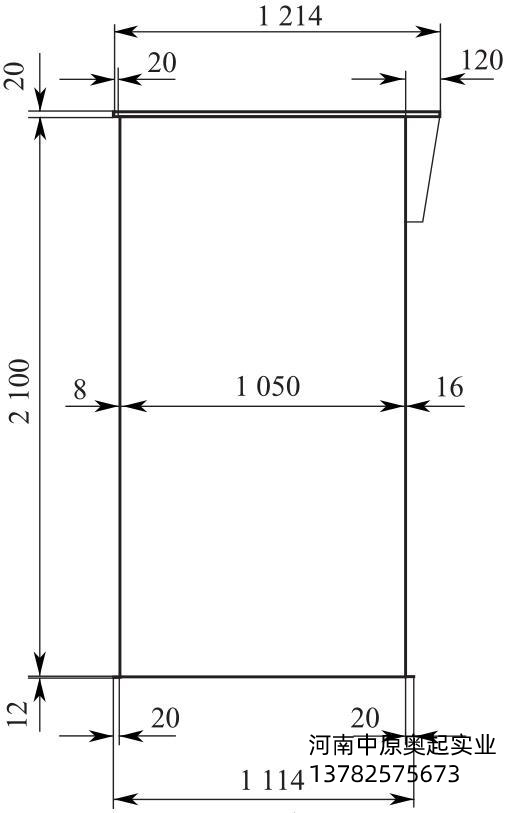全国统一服务热线:13782575673

# 龙门吊大车的计算实例

### 来源：未知   发布时间：2021-03-19 10:33  点击量：

龙门吊双小车起重机，主小车最大额定起重量Q1=100t，自重G1x=18.5t，副小车最大额定起重量Q2=50t，自重G2x=5.6t，主小车可以单独吊重工作，也可和副小车均匀抬吊工作，其最大额定起重量=100t，主副小车吊钩中心线的最小距离b=4m，跨度S=33m，起升高度H=24m，工作级别=A5，主、副小车全部采用国产配置，起升和运行速度在此省略参数，设计大车主梁。

(1)按双小车设计大车主梁

①取起升冲击系数φ2=1.24，运行冲击系数φ4=1.26;其他相关参数为：[β]=700;[σ]=1.40×105kN/m2;E=2.1×108kN/m2;γ=78.5kN/m3;λ=0.2;α=13;αf=13;初取δ=16+8=24mm。

②C1、C2、C3的求值。

R1=(Φ2ξ1+Φ4G1x)/2=(1.24×1000×0.5+1.26×185)/2=426.6kNR2=(Φ2ξ2+Φ4G2x)/2=(1.24×1000×0.5+1.26×56)/2=345.3kN用工程产品设计简化公式③求判别起重量[Q1+Q2]。刚度是控制条件。

取梁净高h0=2.0m=2000mm

取腹板净宽B0=1000mm，主梁设置专用走台。主梁截面设计尺寸见图4-60，省略主梁盖板、腹板加强筋板。

(2)按单小车设计大车主梁

①计算主梁梁高

主梁采用Q235B材料，主梁梁高计算为

[Q]

根据强度控制梁高的公式计算梁高，需先求出K1。

圆整取hq=2200mm。

②初步确定主梁截面面积

取经济梁高盖板与腹板截面积之比￼，上盖板δ3=20mm，下盖板δ4=12mm;

上盖板的截面积尺寸￼

上盖板的宽度￼

取by=1100mm，作为主腹板和副腹板之间的净宽距离，见图4-61。

(3)改用Q345-B材料设计主梁截面

强度为控制条件。

④求强度控制条件下的梁高h强。

取梁净高h0=2.1m=2100mm

⑤设计主梁截面。

取上下盖板的平均厚度为：δP=(δ3+δ4)/2=(20+12)/2=16mm;取腹板净宽B0=1050mm，主梁设置专用走台。

主梁截面设计尺寸见图4-59，省略主梁盖板、腹板加强筋板。

⑥改用Q345-B材料设计主梁截面。刚度是控制条件。取梁净高h0=2.0m=2000mm取腹板净宽B0=1000mm，主梁设置专用走台。

主梁截面设计尺寸见图4-62，省略主梁盖板、腹板加强筋板。

3.结论

由于篇幅所限，根据大量实践设计数据说明，在同参数情况下，双小车与单小车的主梁截面并不都相等，可能要小，也可能要大。与双小车主副小车的吊距、主副小车的起重量、主梁的材料、主梁结构的工作级别、主副小车的起升冲击系数φ2和运行冲击系数φ4的取值大小有关。无论是双小车的何种工况，都推荐按本章双小车的主梁计算介绍设计。

关键词：龙门吊